# Texas Go Math Grade 1 Unit 2 Answer Key Number and Operations: Addition and Subtraction Strategies, Money

Refer to our Texas Go Math Grade 1 Answer Key Pdf to score good marks in the exams. Test yourself by practicing the problems from Texas Go Math Grade 1 Unit 2 Answer Key Number and Operations: Addition and Subtraction Strategies, Money.

## Texas Go Math Grade 1 Unit 2 Answer Key Number and Operations: Addition and Subtraction Strategies, Money

Show What You Knów
Check your understanding of important skills.

Model Subtraction

Use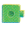to show each number.
Take away. Write how many are left.

Question 1.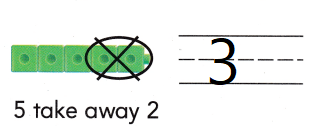Explanation:
The difference of 5 and 2 is 3

Question 2.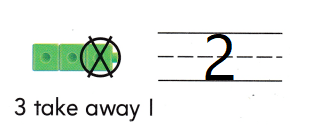Explanation:
The difference of 3 and 1 = 2

Use Pictures to Subtract

Use the picture. Write the subtraction sentence.

Question 3.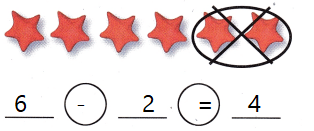Explanation:
The difference of 6 and 2 is 4

Question 4.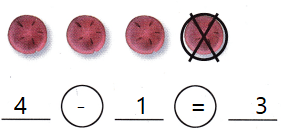Explanation:
The difference of 4 and 1 is 3

Use Symbols to Subtract

Write how many are left.

Question 5.Explanation:
The difference of 3 and 0 is 3

Question 6.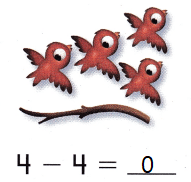Explanation:
The difference of 4 and 4 is 0

Family Note:

Vocabulary Builder

Visualize it

Complete the chart. Mark each row with a ✓.Explanation:
The kid answered as the chart shows

Understand Vocabulary

Complete the sentences with review words.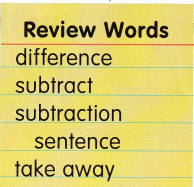Question 1.
3 is the ___ for 5 – 2 = 3.
Explanation:
The difference of 5 and 2 is 3

Question 2.
7 – 4 = 3 is a ___.
Explanation:
7 -4 = 3 is a subtraction sentence

Question 3.
You ___ to solve 5 – 1.
Explanation:
you have to take away 4 from 5 to get 1

Question 4.
You can ___ 2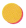from 6.
Explanation:
we can subtract 2 from 6
6 – 2 = 4

Friendship Soup
written by Mary Crobles
illustrated by Chris Simpson
This Take-Home Book belongs to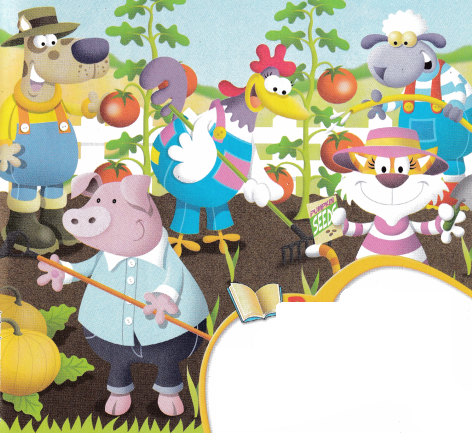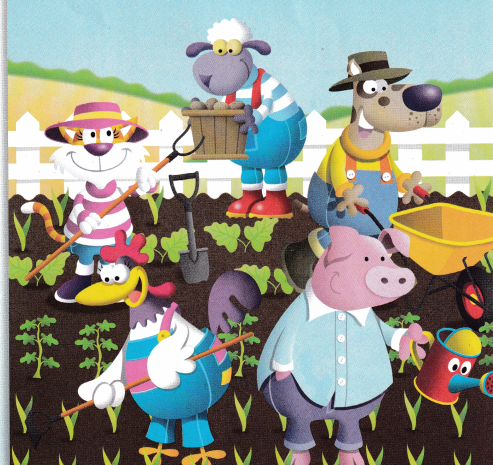All summer the friends worked hard Growing yummy vegetables to eat. Planting, hoeing, watering, weeding Keeping garden rows nice and neat.“Look at this,” cried Hen, seeing her tomatoes.
“Two on the left side and two on the right. Such beautiful tomatoes!
Shall I pick them? I might!”
___ + ___ = ____ tomatoes
2 + 2 = 4 Tomatoes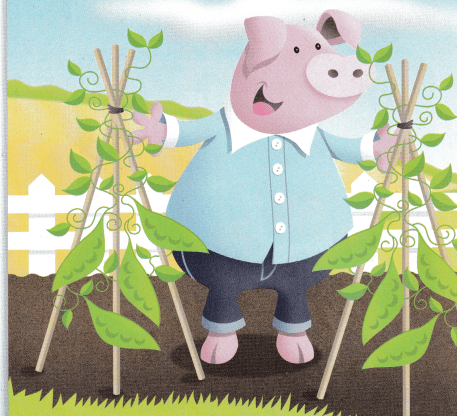“Oh my,” cried Pig.
A week ago they were so little,
But look! They’ve grown so quick!”

How many beans on each plant?
How many in all?
____ + ____ = ____ in all
4 + 4 = 8 in allSheep dug up two potato plants,
“My potatoes look just right.
I dug up two hills of potatoes
And what a beautiful sight!”
____ + ____ = ____ in all
5 + 5 = 10 potatoes“Oh my!” said Dog.
“Onions, big and round.
I don’t think nicer onions
Could be pulled out from the ground!”
___ + ___ = ___ in all
3 + 2 = 5 OnionsCat was the last to notice
The garden had grown wide and tall.
She marveled to see orange pumpkins
Were the biggest veggie of all!

How many big and small pumpkins?
___ + -__ = ___ in all
6 + 6 = 12
6 big and 6 small pumpkins total = 12Here are some of the many things the friends grew in their friendship garden. Color ¡n some of the groups of vegetables. Write an addition sentence to tell how you would add the groups.
___ + ___ = ___
10 + 7 = 17
Pees and tomatoes as one group
Pumpkins and potatoes as the second group
There are 17 in all

Write Math Look at the picture. Draw and write your own story about the number of friends and their tools.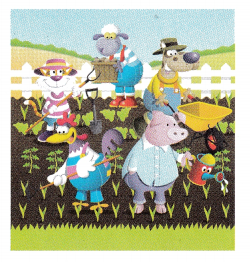Explanation:
There 5 friends and 5 tools
Each friend has 1 tool
5 + 5 = 10

How Many Vegetables?

Look at the picture. Complete the addition sentences.Question 1.
How many pumpkins and onions are there?
__ + ___ = ____
3 + 2 = 5
Explanation:
Three pumpkins and 2 onions total is 5

Question 2.
How many tomatoes and potatoes are there?
__ + ___ = ____
4 + 5 = 9
Explanation:
4 tomatoes and 5 potatoes 9 in all

Question 3.
How many onions and tomatoes are there?
__ + ___ = ____